Question

# **This is for the C Language. Trigonometric Calculator Specification You are required to write a program...

**This is for the C Language.

Trigonometric Calculator

Specification

You are required to write a program that calculates and displays values of the trigonometric functions sine, cosine, and tangent for user-supplied angular values. The basic program must comply with the following specification.

1. When the program is run, it is to display a short welcome message.

```TRIG: the trigonometric calculator
```

2. The program shall display a message prompting the user to enter input from the keyboard.

```Please input request (h-help, q-quit):
```

3. The following help message shall be displayed whenever the user types the letter h at the input prompt.

```The input format is a single line of letters and numbers comprising the
following fields:

These fields must be input in the order shown above, where
is a set of letters, and the others are single numbers defining
the range of computation.

The  field consists of zero or more of the letters from the set
, which indicate respectively,

(1) The desired trig functions: sin, tan, cos.
(2) Whether to use degrees or radians for input parameters.
(3) Whether to quit the program.

Example input: scr 0 2.3 3
```

4. The program shall exit if the user types the letter q at the input prompt.

5. The user may enter a single line of input composed of four fields separated by whitespace,

```
```

where the angle brackets < > indicate particular information fields as described below.

6. The field shall be zero or more of the letters stcdr, indicating sine (s), tangent (t), cosine (c), degrees (d), or radians (r) to indicate trigonometric function types and input units.

7. The field shall be followed by three numerical values that indicate, in order, the starting value for the calculations, the final value for the calculations and the number of intermediate rows in the table. The first two values are floating point, the third is an integer.

8. The following defaults shall be implemented when all or part of the field is missing:

(a) If no function type is specified, all 3 trigonometric functions shall be displayed.

(b) If no unit type is specified, units of entry shall default to degrees.

9. The program shall respond to all inputs in a case insensitive manner. i.e. any letters are valid in both upper and lower case.

10. The program shall validate user input, and recover gracefully from illegal input. Since a user might enter any arbitrary string of characters, many different errors are possible, including:

Specifying both d and r in the field.

Entering invalid letters in the field.

Entering letters or symbols instead of numbers in any of the last three fields.

Failing to enter exactly three numerical values after the field.

Entering a negative or non-integer value for the number of intermediate rows.

11. On detection of illegal input, the following error message shall be displayed:

```Error: Illegal input!
```

12. The results shall be displayed in a tabular format, with the first column containing the angle values in degrees, the second column containing the angle values in radians and the next columns containing the values of the requested trigonometric functions. The values in each row shall be space delimited, with columns 10 characters wide and right justified.
Values in the table shall be displayed to 3 decimal places.
Undefined values shall be represented with the string "N/A".

13. The first row of the table shall display headings describing the contents of the columns.

```   Degrees   Radians       Sin       Cos       Tan
```

The headings for degrees and radians shall always be shown, but those for sin, cos and tan are only displayed if the functions are selected. There shall be a blank line after the header row.

14. After displaying the tabular output or an error message, the program shall return to the input prompt (see Item 2 above).

Example Output

```TRIG: the trigonometric calculator

Please input request (h-help, q-quit): scr 0 2.3 3

0.000     0.000     0.000     1.000
32.945     0.575     0.544     0.839
65.890     1.150     0.913     0.408
98.835     1.725     0.988    -0.154
131.780     2.300     0.746    -0.666

Please input request (h-help, q-quit): q
```

Here is a code for above scenario:

#include <stdio.h>

#include <string>

#include <iostream>

#include <cmath>

#include <algorithm>

#include <fstream>

using namespace std;

void welcome();

float decision(string);

float sides(float &, float &, float &, float &, float &);

int main(int argc, char *argv[])

{

welcome();

return 0;

}

void welcome(){

float valuea;

float valueb;

float valuec;

float valueao;

float valuebo;

float valueco;

cout << "Trig Calculator" << endl << endl;

cout << "Select problem type\n1. Right angle triangle" << endl;

case 1:

cout << "If you do not know the value use 0.\n\n";

valuea = decision("Do you know side A?\n");

valueb = decision("Do you know side B?\n");

valuec = decision("Do you know side C?\n");

valueao = decision("Do you know angle A?\n");

valuebo = decision("Do you know angle B?\n");

sides(valuea, valueb, valuec, valueao, valuebo);

cout << "\n\nTriangle calculated:\n\n";

cout << "Side A: " << valuea << endl;

cout << "Side B: " << valueb << endl;

cout << "Side C: " << valuec << endl;

cout << "Angle A: " << valueao << endl;

cout << "Angle B: " << valuebo << endl << endl;

welcome();

}

}

float decision(string question){

float value;

cout << question;

cin >> value;

return value;

}

float sides(float &a, float &b, float &c, float &ao, float &bo){

if(a !=0 && b!=0 && c==0){

c = sqrt(a * a + b * b);

}

if(a !=0 && c!=0 && b==0){

b = sqrt(c * c - a * a);

}

if(a ==0 && c!=0 && b!=0){

a = sqrt(c * c - b * b);

}

//TAN with side A

if(a!= 0 && b==0 && c==0){

if(ao !=0){

if(bo == 0){

bo = 90-ao;

}

}

if(bo !=0){

if(ao == 0){

ao = 90-bo;

}

}

c = sqrt((a*a)+(b*b));

}

//tan with sideB

if(a == 0 && b!=0 && c==0){

if(ao !=0){

if(bo == 0){

bo = 90-ao;

}

}

if(bo !=0){

if(ao == 0){

ao = 90-bo;

}

}

c = sqrt((a*a)+(b*b));

}

//sin with sideC

if(a == 0 && b==0 && c!=0){

if(ao !=0){

if(bo == 0){

bo = 90-ao;

}

}

if(bo !=0){

if(ao == 0){

ao = 90-bo;

}

}

b = sqrt((c*c)+(a*a));

}

if(ao == 0 && bo ==0){

ao = atan(a/b)*180/3.141592654;

bo = 90 - ao;

}

}

#### Earn Coins

Coins can be redeemed for fabulous gifts.

Similar Homework Help Questions
• ### this is a C++ program! You will write a program that performs the following tasks for...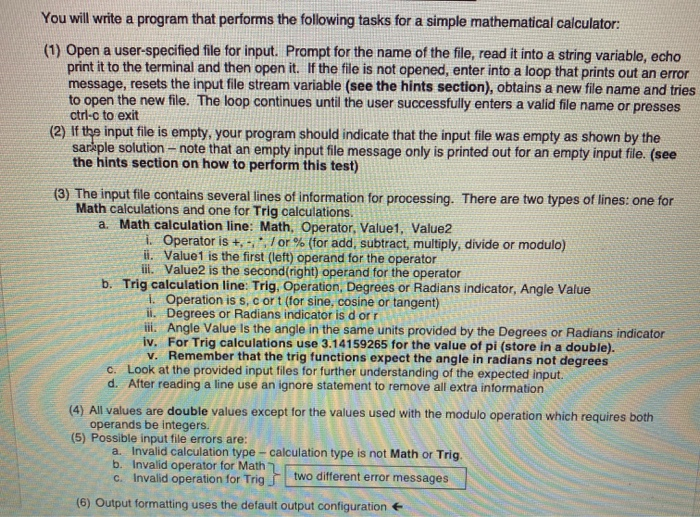this is a C++ program! You will write a program that performs the following tasks for a simple mathematical calculator: (1) Open a user-specified file for input. Prompt for the name of the file, read it into a string variable, echo print it to the terminal and then open it. If the file is not opened, enter into a loop that prints out an error message, resets the input file stream variable (see the hints section), obtains a new file...

• ### Use a switch statement to build a menu-driven calculator

ENGR 40 PROGRAMMING ASSIGNMENT MENU-DRIVEN CALCULATOR PROGRAM Use a switch statement to build a menu-driven calculator. Your program should ask the user to choose from a list of ten possible functions (square root, square, natural log, common log, e'x yx, 1/x, sin(x), cos(x), and tan(x). Each choice will correspond to an integer -example: (1) square root, (2) square, etec. Once the person has input a function choice then a switch statement will control the flow of the program- ie. the program...

• ### a.) Write a C++ program that calculates the value of the series sin x and cos...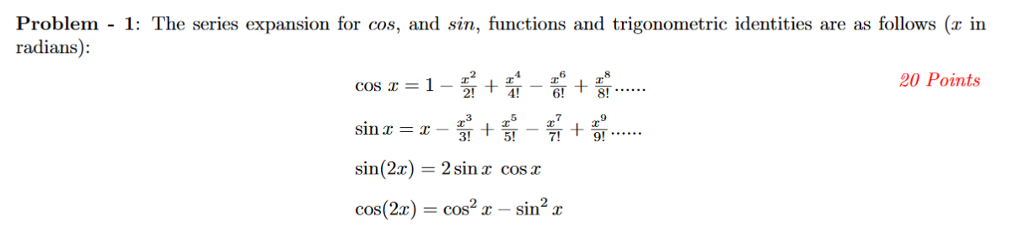a.) Write a C++ program that calculates the value of the series sin x and cos x, sin 2x or cos 2x where the user enters the value of x (in degrees) and n the number of terms in the series. For example, if n= 5, the program should calculate the sum of 5 terms in the series for a given values of x. The program should use switch statements to determine the choice sin x AND cos x, sin...

• ### I need a simple program in C language that calculates the values of Sine, Cosine, and...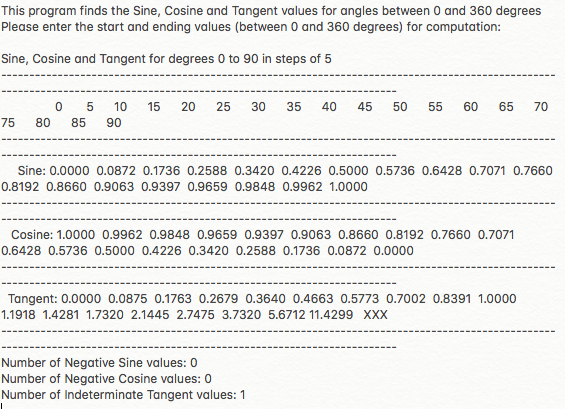I need a simple program in C language that calculates the values of Sine, Cosine, and Tangent of values given in degrees. It has to be a range of values between 0 and 360(integer values). The output should be a table of values showing Sin, Cos and Tan values. The complete computation and printing of the table must be done inside a function. The function also returns to the main program with 3 output arguments: It also needs to show...

• ### In this program, you will be using C++ programming constructs, such as overloaded functions. Write a...

In this program, you will be using C++ programming constructs, such as overloaded functions. Write a program that requests 3 integers from the user and displays the largest one entered. Your program will then request 3 characters from the user and display the largest character entered. If the user enters a non-alphabetic character, your program will display an error message. You must fill in the body of main() to prompt the user for input, and call the overloaded function showBiggest()...

• ### The program should be in C programming The law of sines is an equation relating the...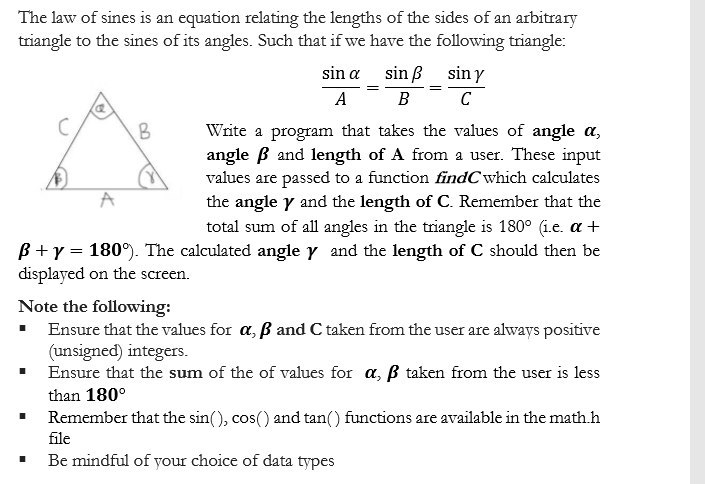The program should be in C programming The law of sines is an equation relating the lengths of the sides of an arbitrary triangle to the sines of its angles. Such that if we have the following triangle: sin a sin ß sin y A B C B Write a program that takes the values of angle a, angle B and length of A from a user. These input values are passed to a function find which calculates the angle...

• ### Please make program. Don't use cstdlib, iosstream, cmath, math.h I didn't learn about it. Only use...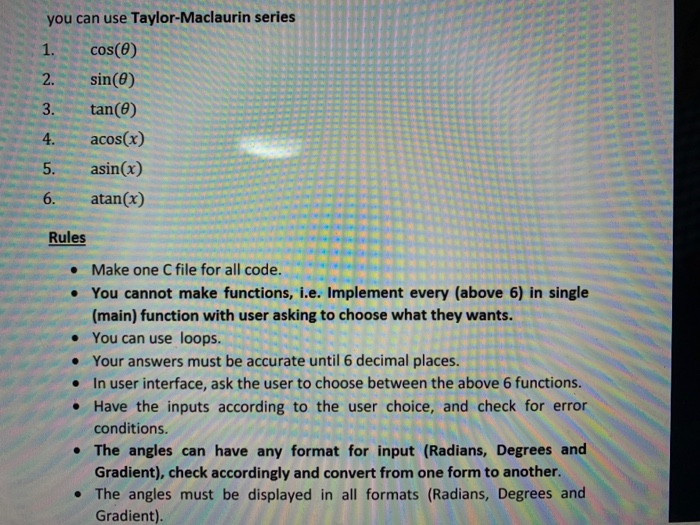Please make program. Don't use cstdlib, iosstream, cmath, math.h I didn't learn about it. Only use for, while if switch function. I learned only those functions. Please comment. Thank you! c++ you can use Taylor-Maclaurin series 1. cos(0) 2. sin(O) 3. tan(e) 4. acos(x) asin(x) 6. atan(x) 5. Rules . • Make one C file for all code. • You cannot make functions, i.e. Implement every (above 6) in single (main) function with user asking to choose what they wants....

• ### It is required to write a Matrix Calculator Program using C Programming Language that could only...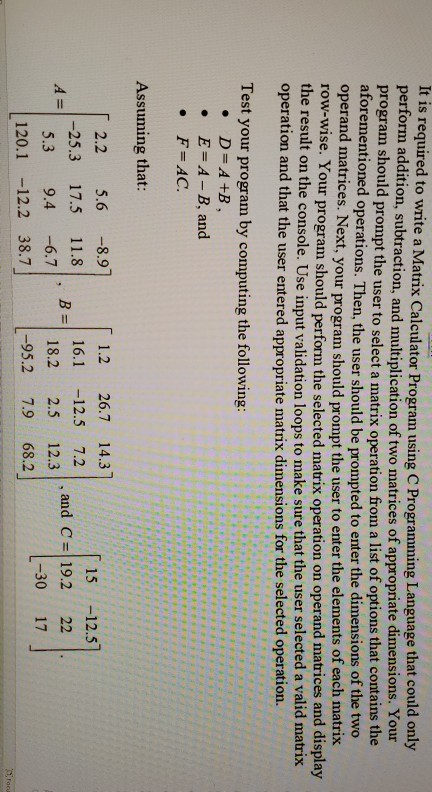It is required to write a Matrix Calculator Program using C Programming Language that could only perform addition, subtraction, and multiplication of two matrices of appropriate dimensions. Your program should prompt the user to select a matrix operation from a list of options that contains the aforementioned operations. Then, the user should be prompted to enter the dimensions of the two operand matrices. Next, your program should prompt the user to enter the elements of each matrix row-wise. Your program...

• ### Program in C++! Thank you in advance! Write a menu based program implementing the following functions: (1) Write a funct...

Program in C++! Thank you in advance! Write a menu based program implementing the following functions: (1) Write a function that prompts the user for the name of a file to output as a text file that will hold a two dimensional array of the long double data type. Have the user specify the number of rows and the number of columns for the two dimensional array. Have the user enter the values for each row and column element in...

• ### Python 3.7 to be used. Just a simple design a program that depends on its own. You should also not need to import anythi...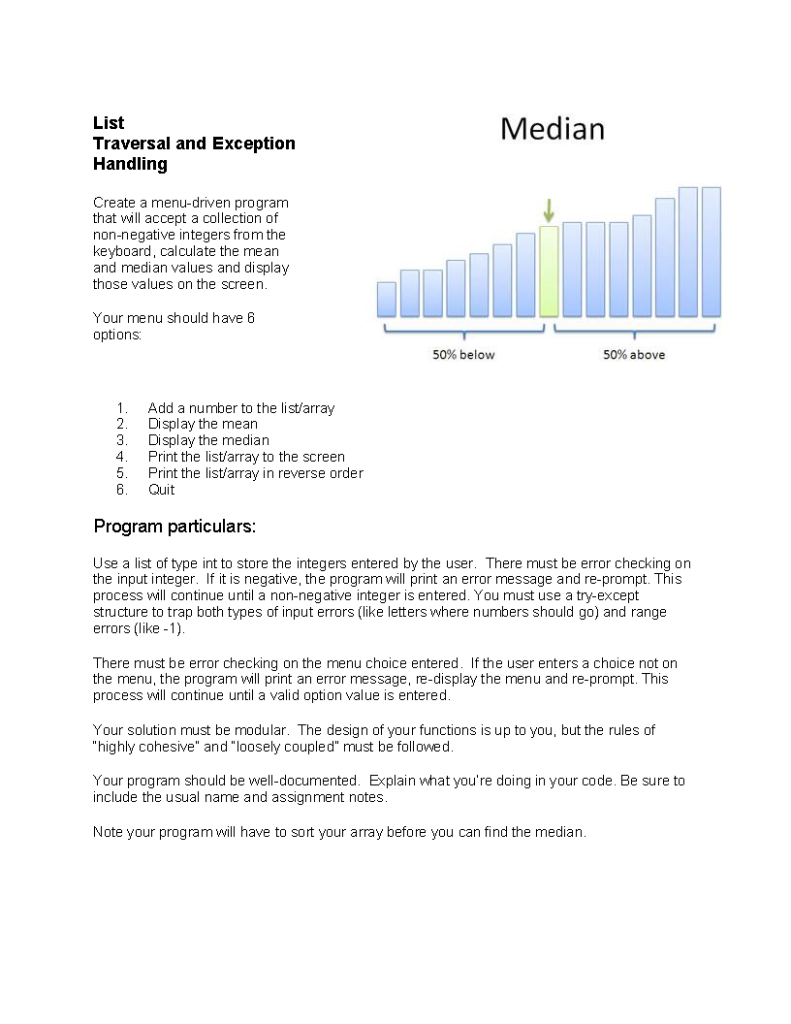Python 3.7 to be used. Just a simple design a program that depends on its own. You should also not need to import anything. No code outside of a function! Median List Traversal and Exception Handling Create a menu-driven program that will accept a collection of non-negative integers from the keyboard, calculate the mean and median values and display those values on the screen. Your menu should have 6 options 50% below 50% above Add a number to the list/array...